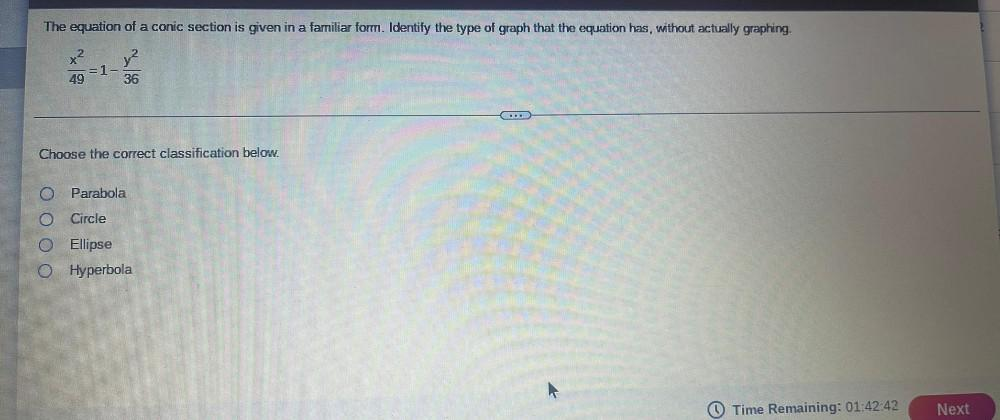Question:

# The equation of a conic section is given in a familiar form. Identify the type of graph that the equation has, without actuallyThe equation of a conic section is given in a familiar form. Identify the type of graph that the equation has, without actually graphing. x2 y2 = 1- 49 36 . Choose the correct classification below. O Parabola Circle O Ellipse 0 Hyperbola Time Remaining: 01:42:42 Next